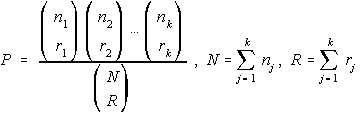HYPERGEO Program

The Hypergeometric Probability Function is given by this program. It asks for two lists (remember: lists must be enclosed in braces {}) of n and r values.

The individual n's must add to be the total number of objects and the individual r's must add to be the total number being chosen.

The probability is returned both as a fraction and a decimal. The numerator of the probability is contained in Nand the denominator in D.

Formulas